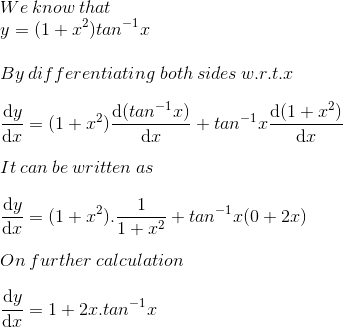# RS Aggarwal Solutions for Class 12 Maths Chapter 10: Differentiation Exercise 10C

Derivatives of inverse trigonometric functions along with various solved examples are the concepts which are covered under the Exercise 10C. To get a clear idea about these concepts, students can solve the exercise using the solutions designed by experts in the respective field. In order to score well in the board exams, students can download RS Aggarwal Solutions Class 12 Maths Chapter 10 Differentiation Exercise 10C free PDF, from the links which are available here.

## RS Aggarwal Solutions for Class 12 Maths Chapter 10: Differentiation Exercise 10C Download PDF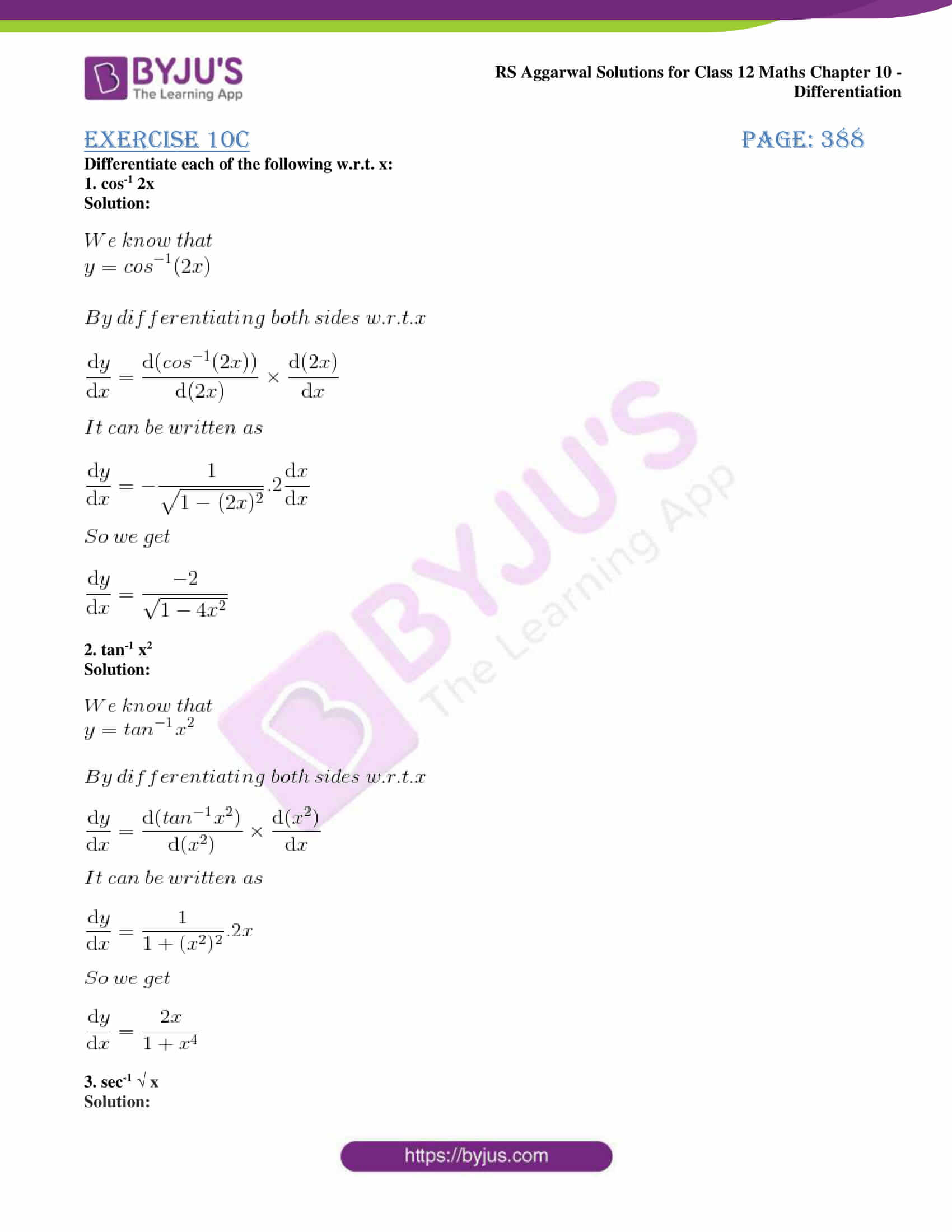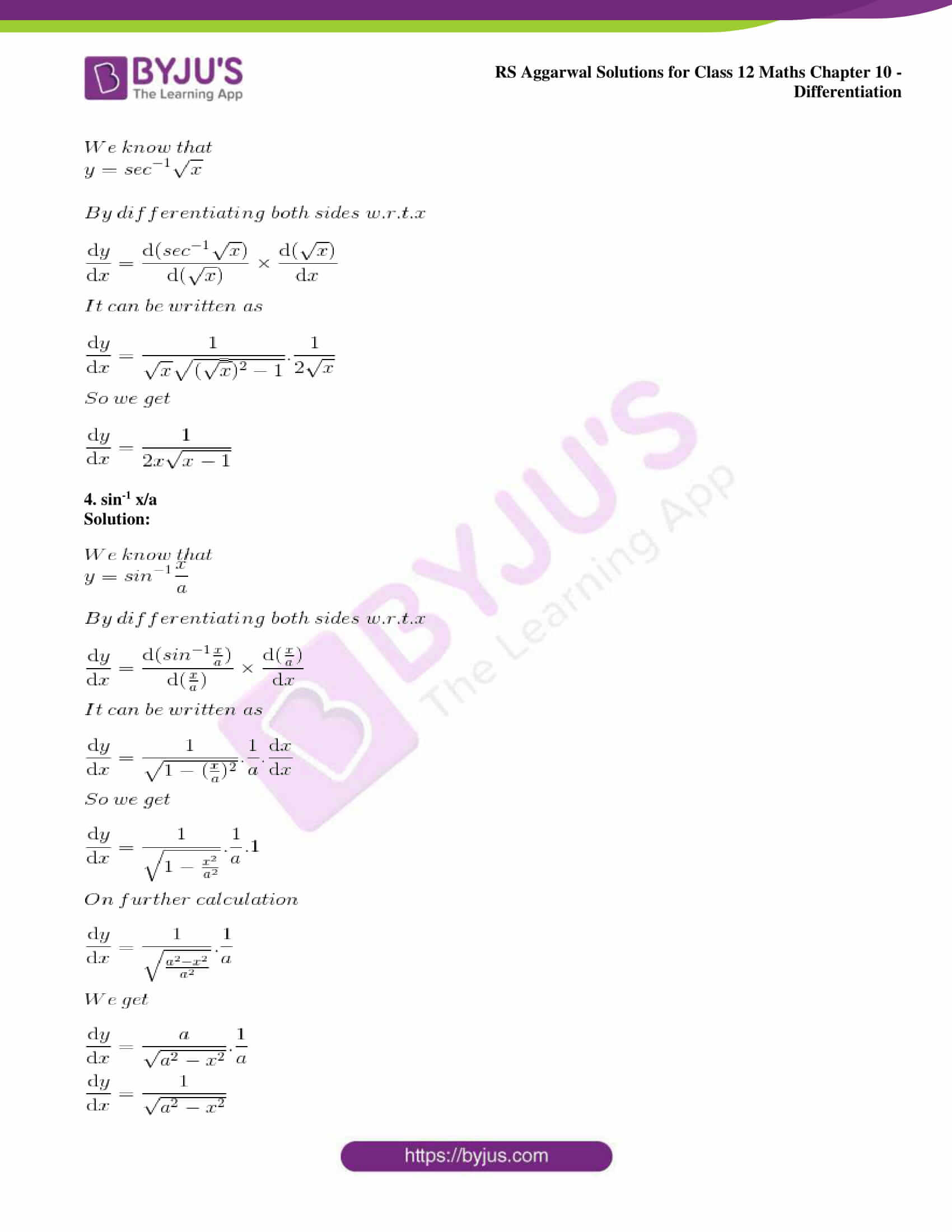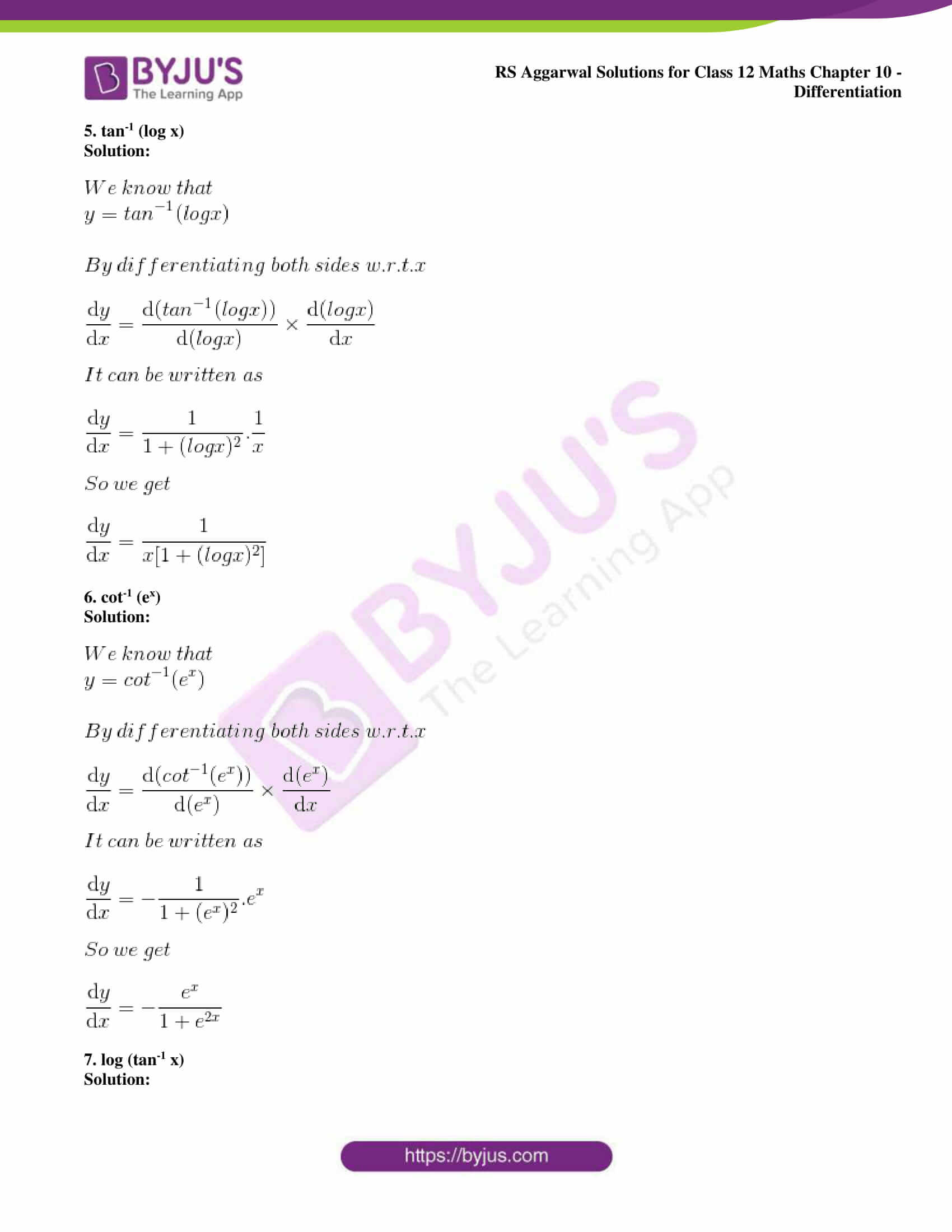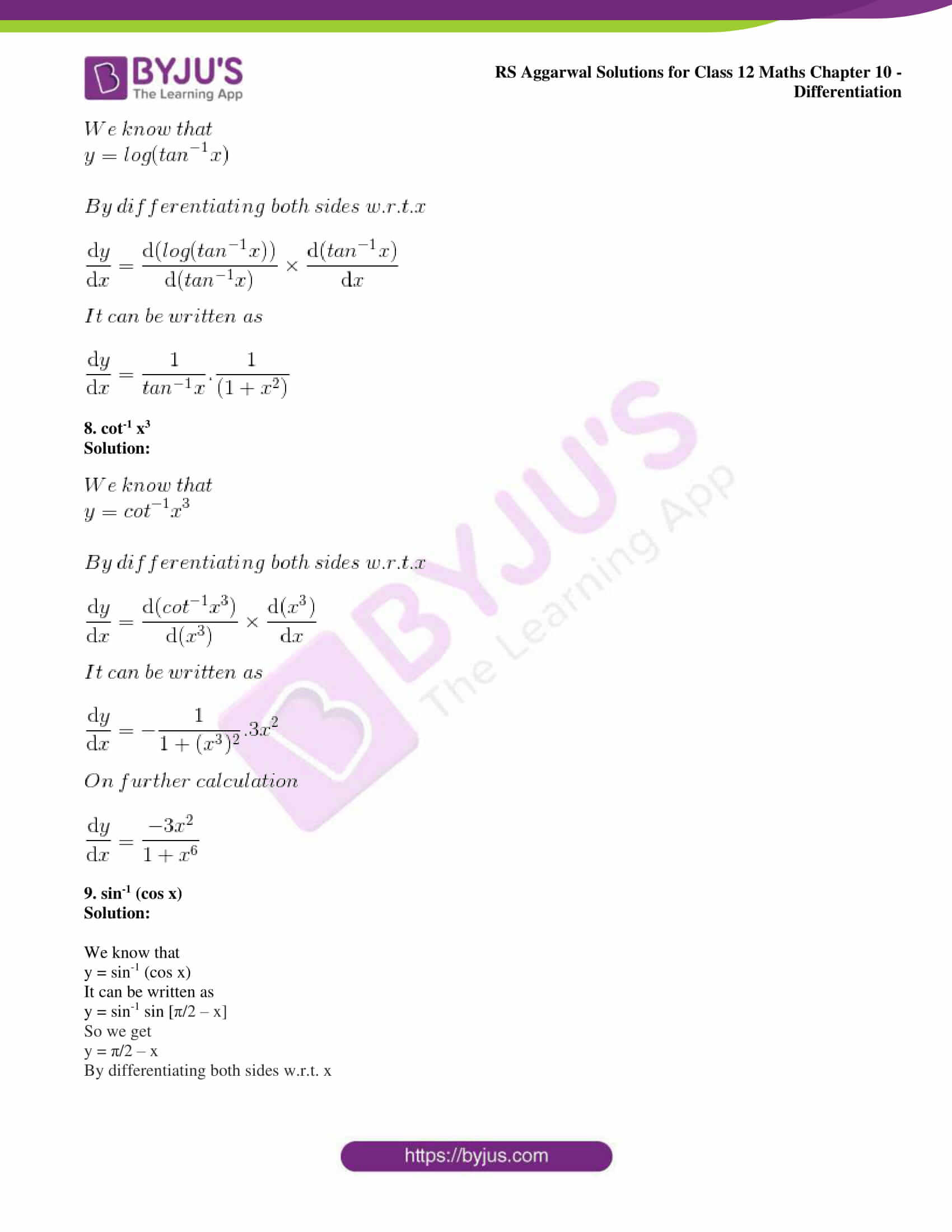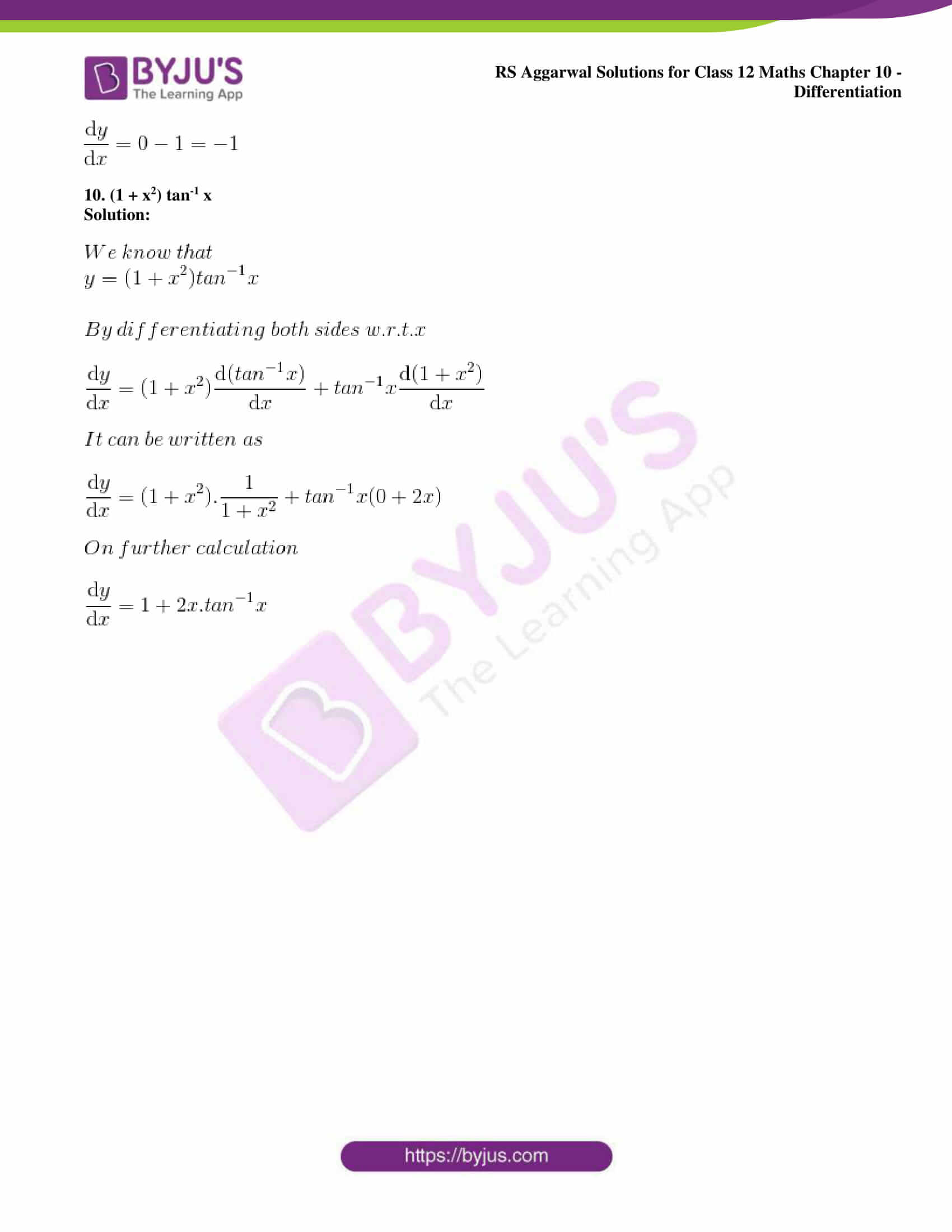### Access other exercise solutions of Class 12 Maths Chapter 10: Differentiation

Exercise 10A Solutions

Exercise 10B Solutions

Exercise 10D Solutions

Exercise 10E Solutions

Exercise 10F Solutions

Exercise 10G Solutions

Exercise 10H Solutions

Exercise 10I Solutions

Exercise 10J Solutions

### Access RS Aggarwal Solutions for Class 12 Maths Chapter 10: Differentiation Exercise 10C

Differentiate each of the following w.r.t. x:

1. cos-1 2x

Solution: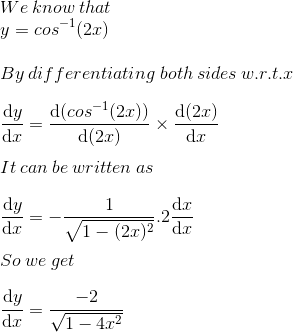2. tan-1 x2

Solution: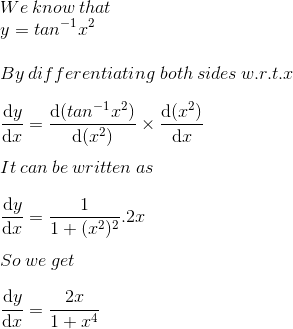3. sec-1 √ x

Solution: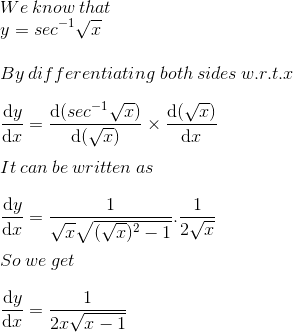4. sin-1 x/a

Solution: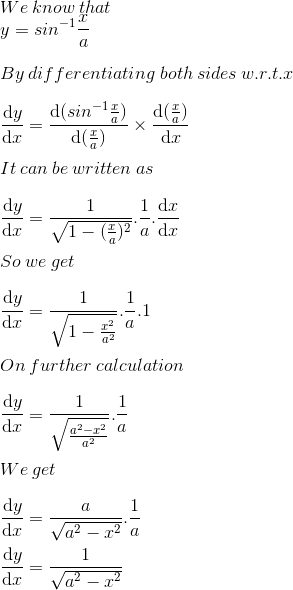5. tan-1 (log x)

Solution: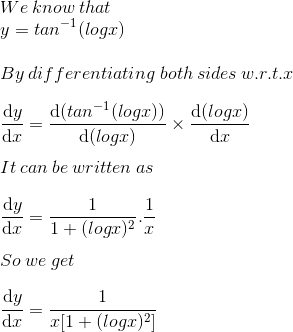6. cot-1 (ex)

Solution: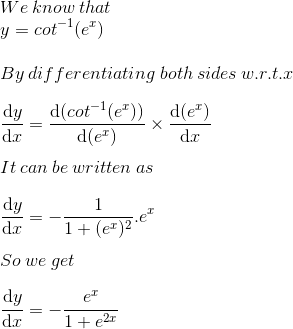7. log (tan-1 x)

Solution: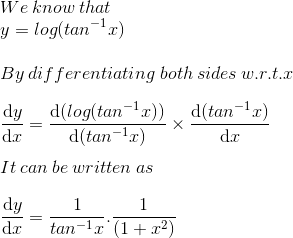8. cot-1 x3

Solution: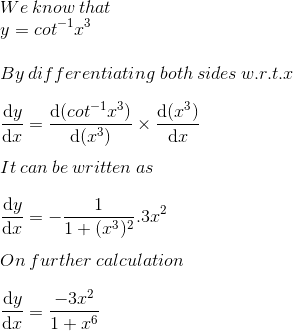9. sin-1 (cos x)

Solution:

We know that

y = sin-1 (cos x)

It can be written as

y = sin-1 sin [π/2 – x]

So we get

y = π/2 – x

By differentiating both sides w.r.t. x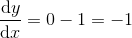10. (1 + x2) tan-1 x

Solution: